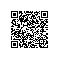# java中的运算符都有哪些

Java提供了丰富的运算符来操纵变量。如果不知道什么是变量，看这里：局部变量、类变量、实例变量有什么区别

## 1、算术运算符## 2、关系运算符## 3、逻辑运算符## 4、位运算符## 5、赋值运算符## 6、其它运算符_条件运算符

variable x = (expression) ? value if true : value if false

"?"号左侧为条件表达式ture或false，如果true则将":"左侧值赋值给"="左侧的变量；如果false则将":"右侧值赋值给"="左侧的变量。

1. public class Test {
2.
3.    public static void main(String args[]){
4.       int a , b;
5.       a = 10;
6.       b = (a == 1) ? 2030;
7.       System.out.println( "Value of b is : " +  b );//结果：Value of b is : 30
8.
9.       b = (a == 10) ? 2030;
10.       System.out.println( "Value of b is : " + b );//结果：Value of b is : 20
11.    }
12. }

## 6、其它运算符_instanceof运算符

instanceof运算符只用于对象引用变量，检查对象是否为特定类型（类或接口类型）。语法：
( Object reference variable ) instanceof (class/interface type)

1. public class Test {
2.
3. public static void main(String args[]){
4. String name = "James";
5. boolean result = name instanceof String;
6. System.out.println(result);//结果：true
7. }
8. }

1. class Vehicle {}
2.
3. public class Car extends Vehicle {
4. public static void main(String args[]){
5. Vehicle a = new Car();
6. boolean result = a instanceof Car;
7. System.out.println(result);//结果：true
8. }
9. }使用钉钉扫一扫加入圈子
+ 订阅